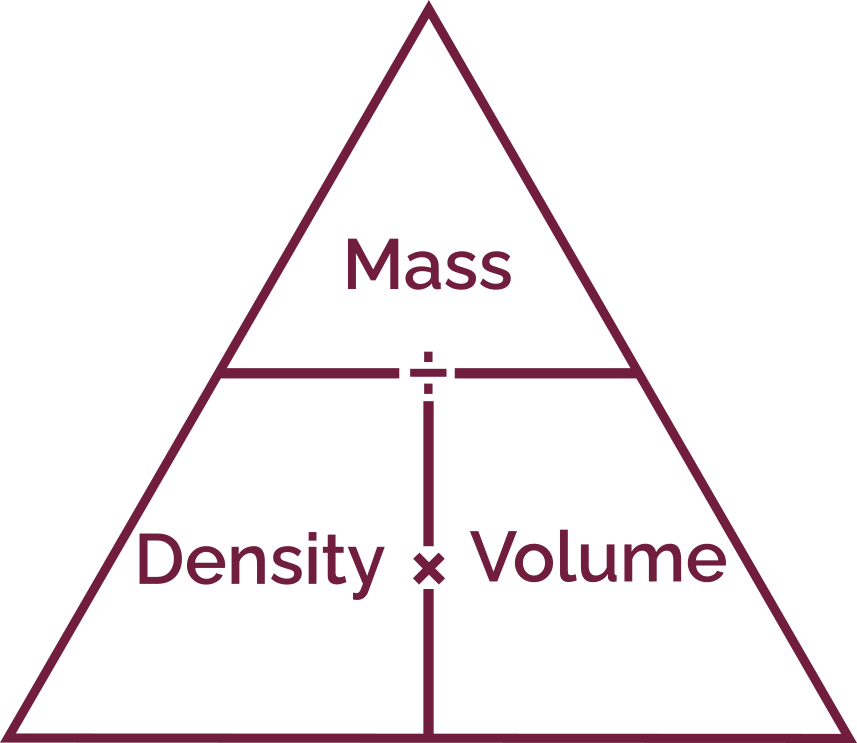# Volume to Mass Calculator

Created by Gabriela Diaz
Last updated: Aug 24, 2022

With the volume to mass calculator, you can instantly convert between volume and mass!

Perhaps you're working on a school assignment, preparing a recipe, or maybe wondering how many cups you need to hold one kilogram of the Universe. In any case, we have you covered!

Keep on reading and also learn:

• How to calculate mass with volume and density;
• How to find volume from mass; and
• The density-mass-volume triangle trick.

## How to calculate mass with volume and density

To calculate mass from volume, we must first know the material's density. Remember that density is a material's property that relates its mass with its volume, telling us how compact is a given substance. Let's take a look at the density formula:

$\footnotesize \text{density} \ (\rho) = \cfrac{\text{mass} \ (m)}{\text{volume} \ (v)}$

Notice that if we want to know the mass, we can easily rearrange the above into:

$\footnotesize \text{mass} \ (m) = \text{volume} \ (v)\ \times \ \text{density} \ (\rho)$

Then, if we know the volume and density of a given material, we can determine the mass by substituting in the expression above. Simple, isn't it? 😎

As you might have already guessed, if what you know are the mass and density, you can also use this mass and volume relationship to obtain the volume instead. In this case, the expression is:

$\footnotesize \text{volume} \ (v) = \cfrac{\text{density} \ (\rho)}{\text{mass} \ (m)}$

As an example, suppose you're preparing a cake, and the recipe calls for a half cup of butter. Do you know how much butter, in mass, you need to buy? Not sure how to find the mass? Let's take a look:

1. We know that butter's density is 959 kg/m3 (check the list of materials in the calculator for this and other substances' density 😉) and that we need half a cup of it. By substituting this information in the mass equation from above:
$\qquad \footnotesize \text{mass} \ (m) = \text{1/2} \ \text{cup} \ \times \ 959 \cfrac{\text{kg}}{\text{m}^3}$
1. To proceed with the calculation, we need to express these amounts in consistent units. Here the conversion factor from cups to cubic meters is 1 cup = 0.0002366 m3:
\qquad \footnotesize \begin{aligned} \text{mass} \ (m) &= \left( \text{1/2} \ \text{cup} \times \cfrac{0.0002366 \ \text{m}^3}{1 \ \text{cup}} \right) \ \times \ 959 \cfrac{\text{kg}}{\text{m}^3} \\ \text{mass} \ (m) &= 0.11344 \ \text{kg} = 113.44 \ \text{g} = 4.002 \ \text{oz} \end{aligned}
1. That's it! Now you can confidently go to the store knowing that you need 113.44 g or 4.002 oz of butter 🧈

You can check more volume and weight units with our volume conversion and weight converter tools!

💡 Water's volume-mass conversion is an easy case to remember. Since its density is 1 kg/L (at 4 °C / 39.2 °F), 1 kilogram of water occupies 1 liter. With this, you can instantly know that 20 L of water weighs 20 kg, 1000 kg occupies 1000 L, and so on.

## Density-mass-volume triangle

You could also opt for using the density-mass-volume triangle! This is a visual trick for remembering the relationship between these three variables.Simply create a triangle and divide it into three sectors, as shown in the figure. The mass is at the very top, the density is on the bottom left, and the volume is on the bottom right. Do you see how the horizontal line looks like a fractional dash?

This way, you won't have to rearrange the density formula, but by looking at the triangle, you can deduce that:

• Density equals mass divided by volume;
• Volume equals mass divided by density; and
• Mass equals density times volume.

## Using the volume to mass calculator

With the volume to mass calculator, you can quickly find the mass of any material or substance. Let's see how to use this tool:

1. First, choose the material that you're interested in. The calculator offers a variety of preloaded materials to select from, including food, metals, gases, or even astronomy-related stuff. Once you have picked it, the calculator will show its Density

If your material is not available, you'll have to manually enter its density.

2. Proceed to input the known volume of your material in the Volume field.

3. Finally, the calculator will show you its Weight/mass.

🙋 You can also use this tool to calculate the volume from a known mass value. Just enter your material's Density and Weight/mass, and the calculator will display its Volume.

Gabriela Diaz
Lookup density or input your own:
Category
Food
Water
Density
lb/cu ft
Input one and get the other:
Volume
cu ft
Weight/mass
lb
People also viewed…

### Cube density

You can determine the density of any cube using our cube density calculator.

### Mohr's circle

Obtain the principal stresses from a plane stress state using our Mohr's circle calculator.